# Sample Problem Boyles Law The gas in a

• Slides: 13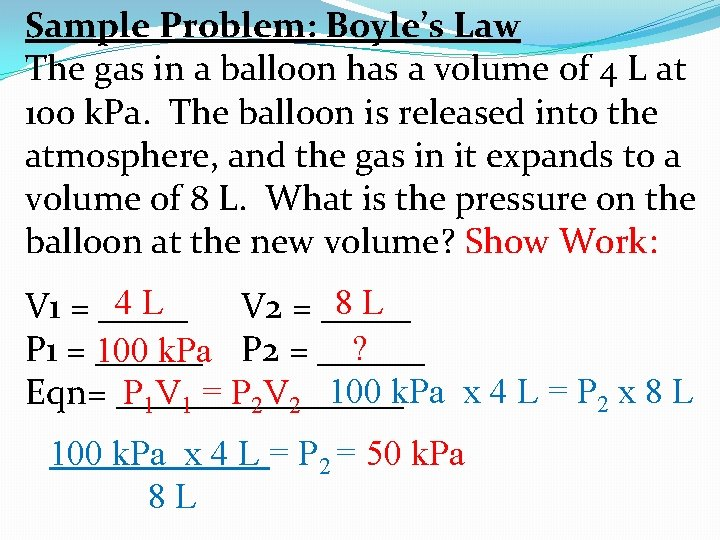Sample Problem: Boyle’s Law The gas in a balloon has a volume of 4 L at 100 k. Pa. The balloon is released into the atmosphere, and the gas in it expands to a volume of 8 L. What is the pressure on the balloon at the new volume? Show Work: 8 L 4 L V 1 = _____ V 2 = _____ ? P 1 = ______ 100 k. Pa P 2 = ______ P 1 V 1 = P 2 V 2 100 k. Pa x 4 L = P 2 x 8 L Eqn= ________ 100 k. Pa x 4 L = P = 50 k. Pa 8 L 2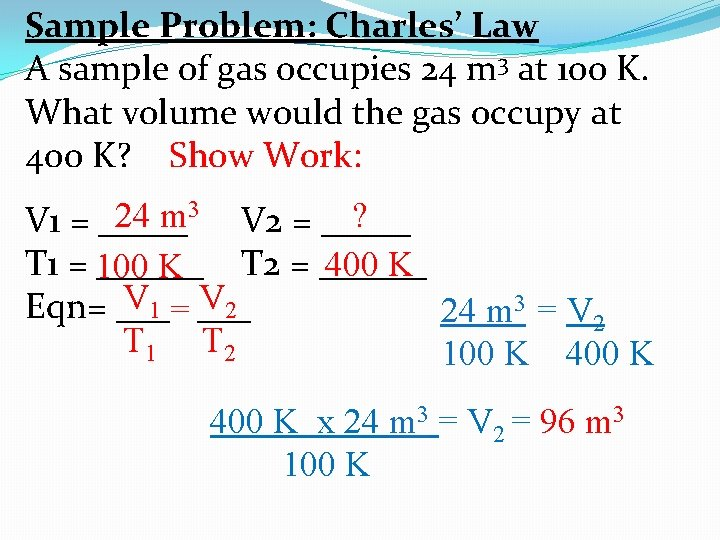Sample Problem: Charles’ Law A sample of gas occupies 24 m 3 at 100 K. What volume would the gas occupy at 400 K? Show Work: 24 m 3 V 2 = _____ ? V 1 = _____ T 1 = ______ T 2 = ______ 400 K 100 K V 1 = V 2 Eqn= ___ 24 m 3 = V 2 T 1 T 2 100 K 400 K x 24 m 3 = V 2 = 96 m 3 100 KSample Problem: Gay-Lussac Law Helium gas is under 1. 12 atm pressure. At 36. 5 o. C, that same gas sample has a pressure of 2. 56 atm. What was the initial temperature of the gas? Show Work: o. C ? 36. 5 T 1 = _____ T 2 = _____ P 1 = ______ 2. 56 atm 1. 12 atm P 2 = ______ P 1 = P 2 1. 12 atm = 2. 56 atm Eqn= ___ T 1 T 2 T 1 309. 5 K 1. 12 atm x 309. 5 K = T 1 = 135. 4 K 2. 56 atm1. Chlorine gas occupies 250 m. L when its pressure is 720 mm Hg. What volume will it occupy when its pressure is 750 mm Hg? V 2 = ? L V 1 = 250 m. L P 2 = 750 mm Hg P 1 = 720 mm Hg P 1 V 1 = P 2 V 2 720 mm Hg x 250 m. L = 750 mm Hg x V 2 720 mm Hg x 250 m. L = V 2 = 240 m. L 750 mm Hg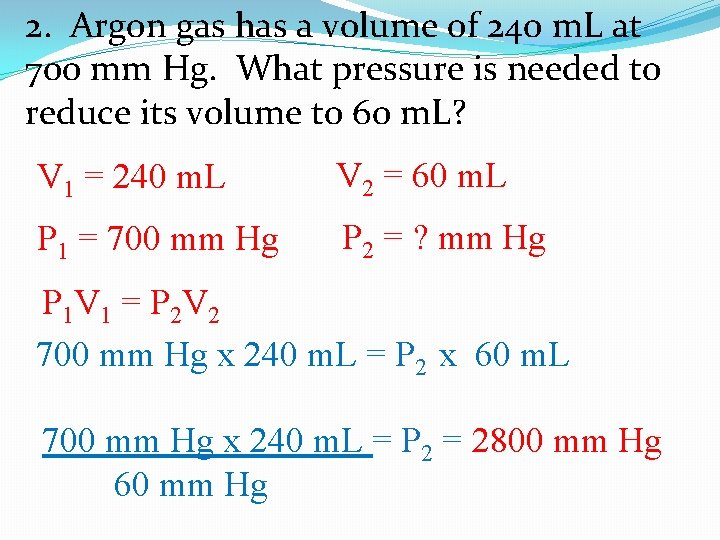2. Argon gas has a volume of 240 m. L at 700 mm Hg. What pressure is needed to reduce its volume to 60 m. L? V 2 = 60 m. L V 1 = 240 m. L P 2 = ? mm Hg P 1 = 700 mm Hg P V =P V 1 1 2 2 700 mm Hg x 240 m. L = P 2 x 60 m. L 700 mm Hg x 240 m. L = P 2 = 2800 mm Hg 60 mm Hg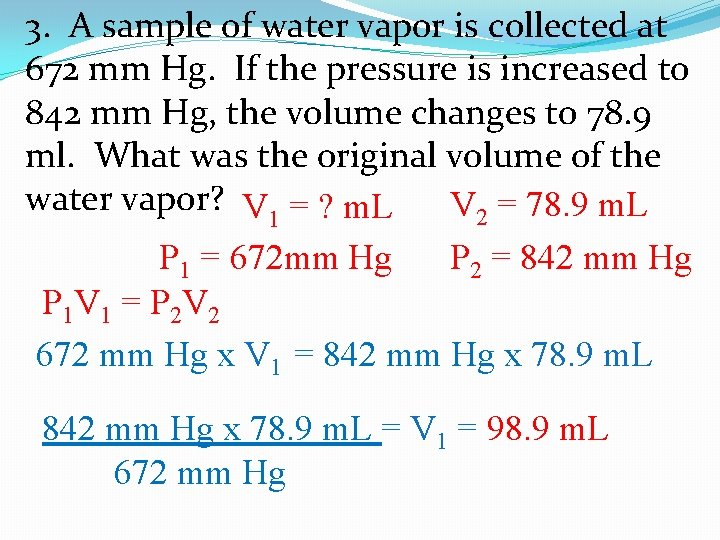3. A sample of water vapor is collected at 672 mm Hg. If the pressure is increased to 842 mm Hg, the volume changes to 78. 9 ml. What was the original volume of the water vapor? V 1 = ? m. L V 2 = 78. 9 m. L P 1 = 672 mm Hg P 2 = 842 mm Hg P 1 V 1 = P 2 V 2 672 mm Hg x V 1 = 842 mm Hg x 78. 9 m. L = V 1 = 98. 9 m. L 672 mm HgConverting from ˚C to K (Remember K = ˚C + 273) Converting from K to ˚C (Remember ˚C = K – 273) 310 37˚C = _____ K 192 465 K = _____ ˚C 275 2˚C = ______ K 0 273 K = ______ ˚C 423 150˚C = _____ K 214 487 K = ______ ˚C 357 84˚C = _____K -91 182 K = ______ ˚C 807 534˚C = _____ K -208 65 K = ______ ˚C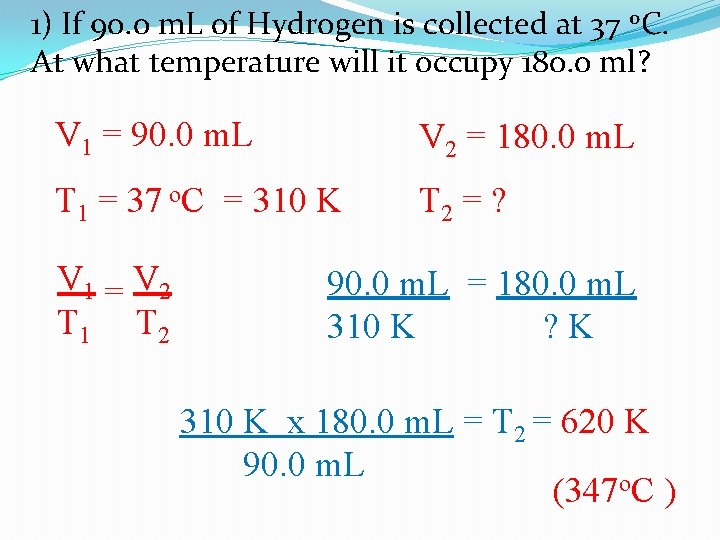1) If 90. 0 m. L of Hydrogen is collected at 37 o. C. At what temperature will it occupy 180. 0 ml? V 1 = 90. 0 m. L V 2 = 180. 0 m. L T 1 = 37 o. C = 310 K T 2 = ? V 1 = V 2 T 1 T 2 90. 0 m. L = 180. 0 m. L 310 K ? K 310 K x 180. 0 m. L = T 2 = 620 K 90. 0 m. L (347 o. C )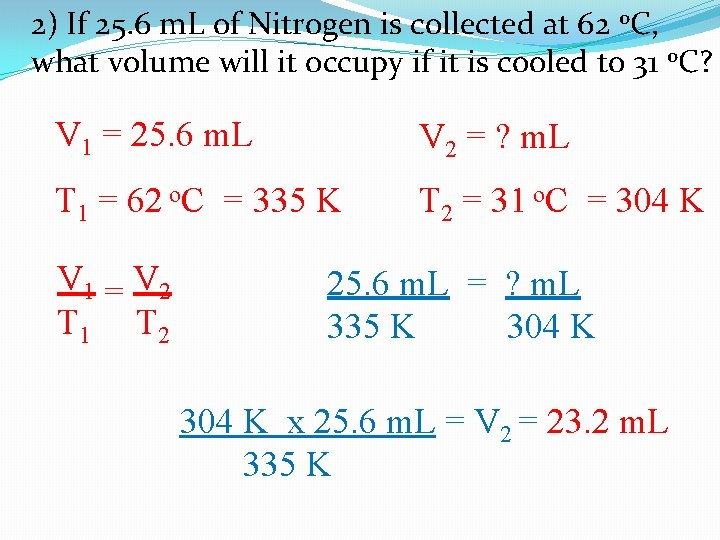2) If 25. 6 m. L of Nitrogen is collected at 62 o. C, what volume will it occupy if it is cooled to 31 o. C? V 1 = 25. 6 m. L V 2 = ? m. L T 1 = 62 o. C = 335 K T 2 = 31 o. C = 304 K V 1 = V 2 T 1 T 2 25. 6 m. L = ? m. L 335 K 304 K x 25. 6 m. L = V 2 = 23. 2 m. L 335 K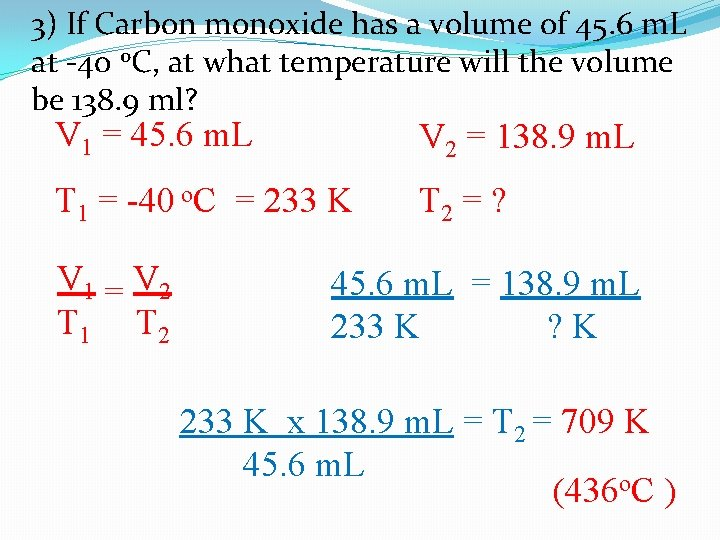3) If Carbon monoxide has a volume of 45. 6 m. L at -40 o. C, at what temperature will the volume be 138. 9 ml? V 1 = 45. 6 m. L V 2 = 138. 9 m. L T 1 = -40 o. C = 233 K T 2 = ? V 1 = V 2 T 1 T 2 45. 6 m. L = 138. 9 m. L 233 K ? K 233 K x 138. 9 m. L = T 2 = 709 K 45. 6 m. L (436 o. C )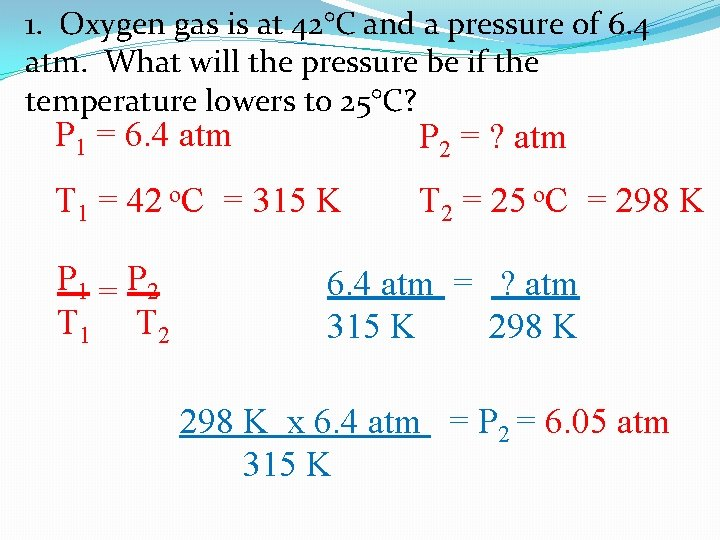1. Oxygen gas is at 42°C and a pressure of 6. 4 atm. What will the pressure be if the temperature lowers to 25°C? P 1 = 6. 4 atm P 2 = ? atm T 1 = 42 o. C = 315 K T 2 = 25 o. C = 298 K P 1 = P 2 T 1 T 2 6. 4 atm = ? atm 315 K 298 K x 6. 4 atm = P 2 = 6. 05 atm 315 K2. Hydrogen gas is under 2. 5 atm pressure. At 55°C, the pressure has changed to 6. 2 atm. What is the initial temperature of the gas? P 1 = 2. 5 atm P 2 = 6. 2 atm T 1 = ? K T 2 = 55 o. C = 328 K P 1 = P 2 T 1 T 2 2. 5 atm = 6. 2 atm ? K 328 K x 2. 5 atm = T 1 = 132 K 6. 2 atm3. The temperature in a greenhouse is 95°C. The door is left open and the temperature decreases to 75°C and the pressure is at 1. 2 atm. What was the initial pressure inside the greenhouse? P 1 = ? atm P 2 = 1. 2 atm T 1 = 95 o. C = 368 K T 2 = 75 o. C = 348 K P 1 = P 2 T 1 T 2 ? atm = 368 K 1. 2 atm 348 K 368 K x 1. 2 atm = P 2 = 1. 27 atm 348 K (1. 3 atm)